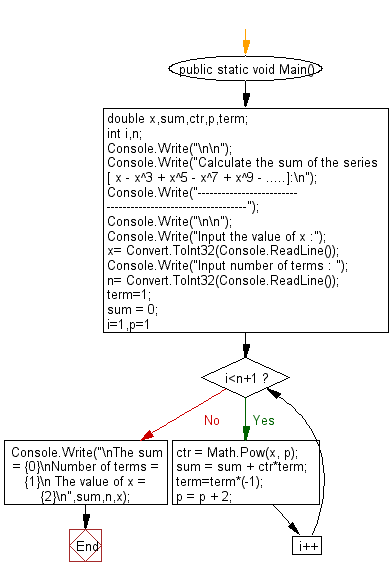﻿ C# - Calculate the sum of the series x - x^3 + x^5 - x^7…# C# Sharp Exercises: Calculate the sum of the series [ x - x^3 + x^5 - x^7 + x^9 -.....]

## C# Sharp For Loop: Exercise-24 with Solution

Write a program in C# Sharp to find the sum of the series [ x - x^3 + x^5 - x^7 + x^9 - ........].

Sample Solution:-

C# Sharp Code:

``````using System;
public class Exercise24
{
public static void Main()
{
double x,sum,ctr,p,term;
int i,n;
Console.Write("\n\n");
Console.Write("Calculate the sum of the series [ x - x^3 + x^5 - x^7 + x^9 - .....]:\n");
Console.Write("------------------------------------------------------------");
Console.Write("\n\n");

Console.Write("Input the value of x :");
Console.Write("Input number of terms : ");
term=1;
sum = 0;
for (i=1,p=1;i<n+1;i++)
{
ctr = Math.Pow(x, p);
sum = sum + ctr*term;
term=term*(-1);
p = p + 2;
}
Console.Write("\nThe sum = {0}\nNumber of terms = {1}\n The value of x = {2}\n",sum,n,x);
}
}
```
```

Sample Output:

```Calculate the sum of the series [ x - x^3 + x^5 - x^7 + x^9 - .....]:
------------------------------------------------------------

Input the value of x :Input number of terms :
The sum = 410
Number of terms = 5
The value of x = 2
```

Flowchart:C# Sharp Code Editor:

Contribute your code and comments through Disqus.

What is the difficulty level of this exercise?

Test your Programming skills with w3resource's quiz.

﻿